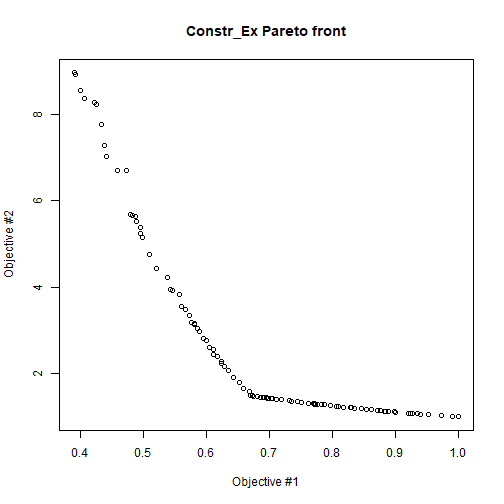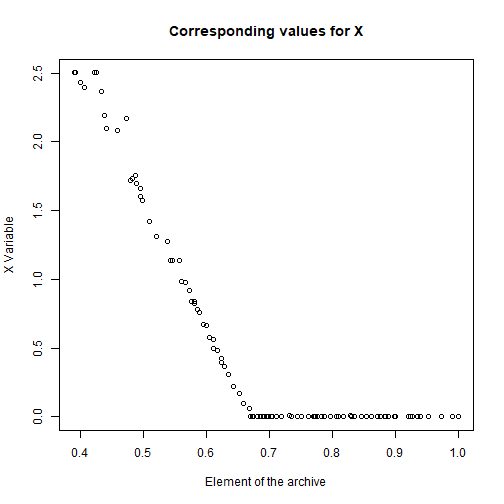# Short Description

caRamel is a multiobjective evolutionary algorithm combining the MEAS algorithm and the NGSA-II algorithm.

Download the package from CRAN or GitHub and then install and load it.

Dealing with constraints is possible with caRamel by returning a NaN value for an infeasible solution. See the example below.

``````library(caRamel)
``````
``````## Loading required package: geometry
``````
``````## Warning: package 'geometry' was built under R version 3.5.3
``````
``````## Loading required package: parallel
``````
``````## Package 'caRamel' version 1.1
``````

# Test functions

## Constr-Ex problem

Constr-Ex test function has two objectives with two variables and two inequality constraints.

``````constr_ex <- function(i) {
# functions f1 and f2
s1 <- x[i,1]
s2 <- (1. + x[i,2]) / x[i,1]
# now test for the feasibility
# constraint g1
if((x[i,2] + 9. * x[i,1] - 6.) < 0. | (-x[i,2] + 9. * x[i,1] -1.) < 0.) {
s1 <- NaN
s2 <- NaN
}
return(c(s1, s2))
}
``````

Note that :

• parameter i is mandatory for the management of parallelism.
• the variable must be named x and is a matrix of size [npopulation, nvariables].

The variable lies in the range [0.1, 1] and [0, 5]:

``````nvar <- 2 # number of variables
bounds <- matrix(data = 0., nrow = nvar, ncol = 2) # upper and lower bounds
bounds[1, 1] <- 0.1
bounds[1, 2] <- 1.
bounds[2, 1] <- 0.
bounds[2, 2] <- 5.
``````

Both functions are to be minimized:

``````nobj <- 2 # number of objectives
minmax <- c(FALSE, FALSE) # min and min
``````

Before calling caRamel in order to optimize the Constr_Ex problem, some algorithmic parameters need to be set:

``````popsize <- 100 # size of the genetic population
archsize <- 100 # size of the archive for the Pareto front
maxrun <- 1000 # maximum number of calls
prec <- matrix(1.e-3, nrow = 1, ncol = nobj) # accuracy for the convergence phase
``````

Then the minimization problem can be launched:

``````results <-
caRamel(nobj,
nvar,
minmax,
bounds,
constr_ex,
popsize,
archsize,
maxrun,
prec,
carallel=FALSE) # no parallelism
``````
``````## Beginning of caRamel optimization <-- Fri May 24 12:15:45 2019
``````
``````## Number of variables : 2
``````
``````## Number of functions : 2
``````
``````## Done in 2.87477993965149 secs --> Fri May 24 12:15:48 2019
``````
``````## Size of the Pareto front : 99
``````
``````## Number of calls : 1000
``````

Test if the convergence is successful:

``````print(results\$success==TRUE)
``````
``````##  TRUE
``````

Plot the Pareto front:

``````plot(results\$objectives[,1], results\$objectives[,2], main="Constr_Ex Pareto front", xlab="Objective #1", ylab="Objective #2")
````````````plot(results\$parameters, main="Corresponding values for X", xlab="Element of the archive", ylab="X Variable")
``````